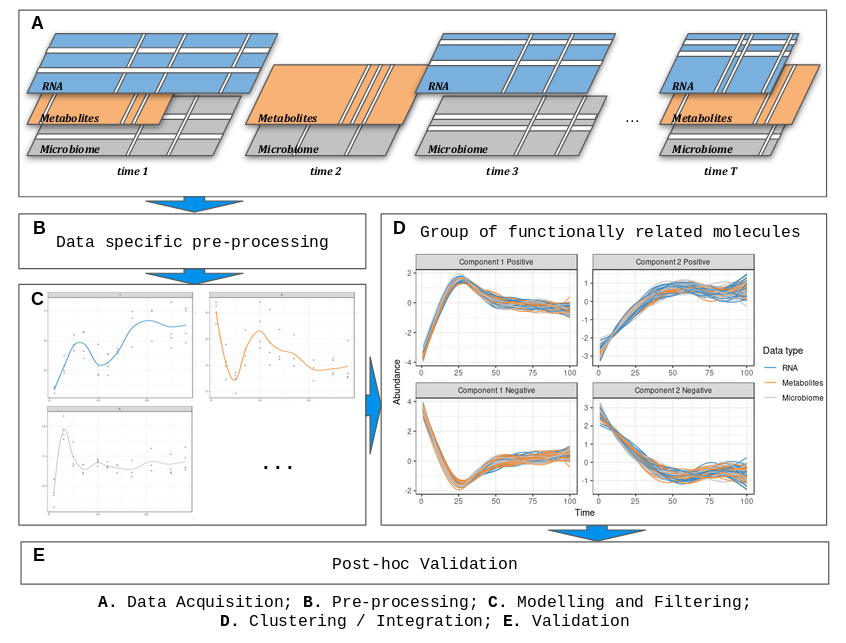# 1 Introduction

timeOmics is a generic data-driven framework to integrate multi-Omics longitudinal data (A.) measured on the same biological samples and select key temporal features with strong associations within the same sample group.

The main steps of timeOmics are:

• a pre-processing step (B.) Normalize and filter low-expressed features, except those not varying over time,
• a modelling step (C.) Capture inter-individual variability in biological/technical replicates and accommodate heterogeneous experimental designs,
• a clustering step (D.) Group features with the same expression profile over time. Feature selection step can also be used to identify a signature per cluster,
• a post-hoc validation step (E.) Ensure clustering quality.

This framework is presented on both single-Omic and multi-Omics situations.Framework Overview

Bodein A, Chapleur O, Droit A and Lê Cao K-A (2019) A Generic Multivariate Framework for the Integration of Microbiome Longitudinal Studies With Other Data Types. Front. Genet. 10:963. doi:10.3389/fgene.2019.00963

# 2 Start

## 2.1 Installation

### 2.1.1 Lastest Bioconductor Release

``````## install BiocManager if not installed
if (!requireNamespace("BiocManager", quietly = TRUE))
install.packages("BiocManager")
## install timeOmics
BiocManager::install('timeOmics')``````

### 2.1.2 Lastest `Github` version

``````install.packages("devtools")
library(devtools)
install_github("abodein/timeOmics")``````

``library(timeOmics)``

## 2.3 Useful package to run this vignette

``library(tidyverse)``

# 3 Required data

Each omics technology produces count or abundance tables with samples in rows and features in columns (genes, proteins, species, …). In multi-Omics, each block has the same rows and a variable number of columns depending on the technology and number of identified features.

We assume each block (omics) is a matrix/data.frame with samples in rows (similar in each block) and features in columns (variable number of column). Normalization steps applied to each block will be covered in the next section.

For this example, we will use a part of simulated data based on the above-mentioned article and generated as follow:

Twenty reference time profiles, were generated on 9 equally spaced* time points and assigned to 4 clusters (5 profiles each). These ground truth profiles were then used to simulate new profiles. The profiles from the 5 individuals were then modelled with `lmms` (Straube et al. 2015). Please check (Bodein et al. 2019) for more details about the simulated data.

To illustrate the filtering step implemented later, we add an extra noisy profile resulting in a matrix of (9x5) x (20+1).

* It is not mandatory to have equally spaced time points in your data.

``````data("timeOmics.simdata")
sim.data <- timeOmics.simdata\$sim

dim(sim.data) ``````
``##  45 21``
``head(sim.data[,1:6])``
``````##            c0       c1.0       c1.1        c1.2      c1.3        c1.4
## A_1 0.6810022 -0.1681427 -0.1336986  0.12040677 0.4460119 -0.93382470
## A_2 1.4789556  0.4309468  1.1172245 -0.08183742 0.4585589 -0.56857351
## A_3 0.9451049  1.4676125  1.6079441 -0.11034711 1.5761445 -0.09178880
## A_4 0.7403461  1.1211525  1.7702314  0.17460753 1.4079393 -0.00414130
## A_5 0.9291161  1.2387863  1.8332048 -0.03780133 1.2714786  0.01158791
## A_6 1.0408472  2.3145195  2.5332477  0.23133263 2.1085377  0.81762482``````

# 4 Data preprocessing

Every analysis starts with a pre-processing step that includes normalization and data cleaning. In longitudinal multi-omics analysis we have a 2-step pre-processing procedure.

## 4.1 Platform-specific

Platform-specific pre-processing is the type of normalization normally used without time component. It may differ depending on the type of technology.

The user can apply normalization steps (log, scale, rle, …) and filtering steps (low count removal, …).

It is also possible to handle microbiome data with Centered Log Ratio transformation as described here.

That is why we let the user apply their favorite method of normalization.

## 4.2 Time-specific

In a longitudinal context, one can be interested only in features that vary over time and filter out molecules with a low variation coefficient.

To do so, we can first naively set a threshold on the variation coefficient and keep those features that exceed the threshold.

``````remove.low.cv <- function(X, cutoff = 0.5){
# var.coef
cv <- unlist(lapply(as.data.frame(X),
function(x) abs(sd(x)/mean(x))))
return(X[,cv > cutoff])
}

data.filtered <- remove.low.cv(sim.data, 0.5)``````

# 5 Time Modelling

The next step is the modelling of each feature (molecule) as a function of time.

We rely on a Linear Mixed Model Splines framework (package `lmms`) to model the features expression as a function of time by taking into account inter-individual variability.

`lmms` fits 4 different types of models described and indexed as below and assigns the best fit for each of the feature.

• 0 = linear model,
• 1 = linear mixed effect model spline (LMMS) with defined basis,
• 2 = LMMS taking subject-specific random intercepts,
• 3 = LMMS with subject-specific intercept and slope.

The package also has an interesting feature for filtering profiles which are not differentially expressed over time, with statistical testing (see `lmms::lmmsDE`).

Once run, `lmms` summarizes each feature into a unique time profile.

## 5.1`lmms` example

`lmms` requires a data.frame with features in columns and samples in rows.

For more information about `lmms` modelling parameters, please check `?lmms::lmmSpline`

``````# numeric vector containing the sample time point information
time <- timeOmics.simdata\$time
``##  1 2 3 4 5 6``
``````lmms.output <- lmms::lmmSpline(data = data.filtered, time = time,
sampleID = rownames(data.filtered), deri = FALSE,
basis = "p-spline", numCores = 4, timePredict = 1:9,
keepModels = TRUE)
modelled.data <- t(slot(lmms.output, 'predSpline'))``````

The `lmms` object provides a list of models for each feature. It also includes the new predicted splines (modelled data) in the `predSpline` slot. The produced table contains features in columns and now, times in rows.

Let’s plot the modelled profiles.

``````# gather data
data.gathered <- modelled.data %>% as.data.frame() %>%
rownames_to_column("time") %>%
mutate(time = as.numeric(time)) %>%
pivot_longer(names_to="feature", values_to = 'value', -time)

# plot profiles
ggplot(data.gathered, aes(x = time, y = value, color = feature)) + geom_line() +
theme_bw() + ggtitle("`lmms` profiles") + ylab("Feature expression") +
xlab("Time")``````